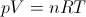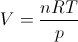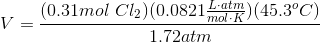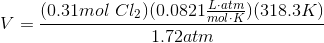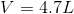# AP Chemistry : Stoichiometry with Reactions

## Example Questions

### Example Question #1 : Stoichiometry With Reactions

Consider the reaction of baking soda and vinegar in an aqueous solution: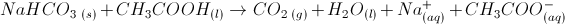If we begin with 17g of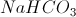and excess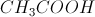, what is the volume of the gas released?

Assume that all gases behave as ideal gases at atmospheric pressure. The temperature is 298K.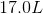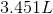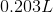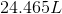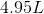Explanation:

We begin with 17 g of, so our first step is to calculate the molecular weight of.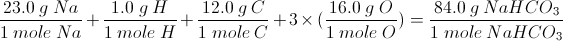Now with the molecular weight, we will calculate the number of moles within 17 g of.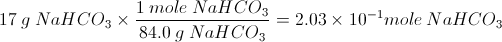Now we will use this to calculate the number of moles of gas produced. In this reaction, the only gas produced is carbon dioxide.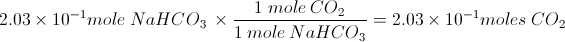An ideal gas at 1 atmosphere and 298 K has the molar volume of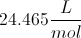.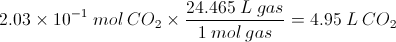### Example Question #2 : Stoichiometry With Reactions

What volume of 0.5M NaOH is necessary to neutralize 15mL of 1.0M nitrous acid?

30L

30mL

10mL

1L

30mL

Explanation:

Both the acid and the base provide one equivalent of the necessary ions (H+ and OH–, respectively) and therefore you can use the equation M1V1 = M2V2.

(1)(15) = (0.5)V2

V2 = 30mL

### Example Question #2 : Stoichiometry With Reactions

What volume of 0.5M Mg(OH)2 is necessary to neutralize 15mL of 1.0M nitrous acid?

1L

15L

15mL

30mL

10mL

30mL

Explanation:

This problem is tricky because you must realize that Mg(OH)2 will release 2 molecules of OH– whenever one dissolves; thus, you only need half the amount of Mg(OH)2 to neutralize all of the nitrous acid, which only gives 1 equivalent.

M1V1 = M2V2

Plugging in the values, you get 30mL, but in reality you only need half of that amount.

### Example Question #3 : Stoichiometry With Reactions

Magnesium will combine with oxygen gas to form magnesium oxide according to the balanced equation below.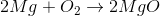If 65g of MgO are created in the reaction, how many grams of magnesium are used in the reaction?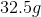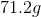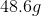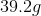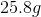Explanation:

In order to determine how many grams of magnesium are used in the reaction, we can use the percentage by mass of magnesium in MgO in order to find out how much magnesium is in 65 grams of MgO. The molar mass of MgO is 40.3 grams. The molar mass of magnesium is 24.3 grams. Dividing 24.3 by 40.3 reveals that magnesium makes up 60% of the mass of MgO.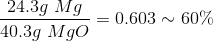Multiplying 65 grams by 0.603 reveals that there are 39.2 grams of magnesium in 65 grams of MgO.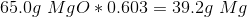An alternative means of solving the problem would be to convert grams of MgO to moles. The ratio of MgO to Mg in the given chemical equation is 2:2, thus the number of moles of MgO formed is equal to the number of moles of Mg reacted. Once this number is found, it can be converted back to grams using the molar mass of magnesium.

### Example Question #4 : Stoichiometry With Reactions

Consider the following chemical reaction: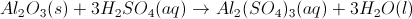How many moles of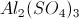will be produced if 75.0mL of water is produced?

There is not enough information to answer this question

4.17mol

1.56 moles

1.39mol

1.39mol

Explanation: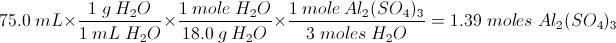This problem is solved based on stoichiometry. Using the molecular weights and ratios in the given reaction, we can solve for the amount ofproduced.

### Example Question #5 : Stoichiometry With Reactions

The formation of ammonia is given by the following equation: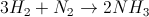Assuming you started with 20g of hydrogen gas, how much nitrogen gas is necessary to react the hydrogen gas?

40g

6.67g

93.33g

60g

93.33g

Explanation:

We can convert the mass of hydrogen from grams to moles by using the equation moles = mass / molar mass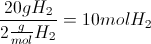Now that we have the moles of hydrogen, we can use the molar ratio given in the equation of 1:3. Since we need 1/3 of the moles of hydrogen as nitrogen, we know we need 3.33 moles of nitrogen gas.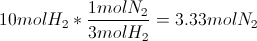Now, we simply multiply the moles by the molar mass of nitrogen gas (28 grams per mol) and we find we need 93.33 grams of nitrogen gas.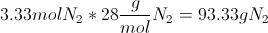### Example Question #6 : Stoichiometry With Reactions

The formation of ammonia is given by the following equation:Assuming you start with 15mol of hydrogen gas, how many moles of nitrogen gas will be needed to completely react the hydrogen gas?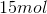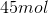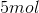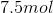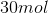Explanation:

Since the amount of hydrogen gas is given in moles, this solution is as easy as using the molar ratio between the reactants. Looking at the equation, we see that there is a 1:3 ratio for nitrogen to hydrogen. As a result, we would need one third of the amount of hydrogen available in order to use up all of the hydrogen gas.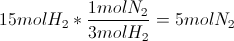### Example Question #8 : Stoichiometry With Reactions

How many moles of water vapor will be formed when 5.00g of hydrogen gas react with excess oxygen?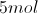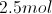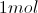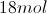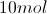Explanation:

When dealing with questions related to stoichiometry, it is important to compare only mole-to-mole ratios.

Hydrogen gas is diatomic. When converting grams to moles, we will need to double the atomic mass of hydrogen.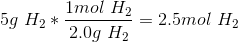We now know we have 2.5 moles of hydrogen gas. Now we need to write a balanced equation to determine the conversion factor between hydrogen gas and the product.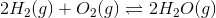For every two moles of hydrogen consumed, two moles of water are produced. Using this ratio and the starting moles of hydrogen, we can calculate the moles of water produced.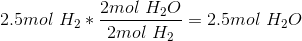### Example Question #1 : Stoichiometry With Reactions

What volume of chlorine gas at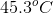is needed to react with 14.2g of sodium to form NaCl at 1.72atm?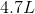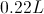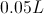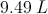There is not enough information to answer this questionExplanation: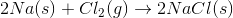First, we need to solve for the moles of chlorine gas needed to react with the full amount of sodium metal.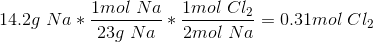Now, using the ideal gas law, we can solve for the volume that this gas would occupy at the given temperature and pressure.